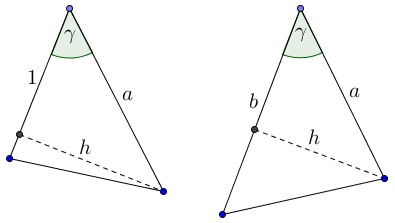# The Law of Cosines for 60° and 120°

In the spirit of Proof #3 of the Pythagorean theorem, we show that

In a triangle $ABC,\;$ with sides $a=BC,\;$ $b=AC,\;$ $c=AB,\;$ and $\gamma=\angle ACB,\;$ we have

$c^2=a^2+b^2-ab\;$ if $\gamma=60^{\circ}\;$ and
$c^2=a^2+b^2+ab\;$ if $\gamma=120^{\circ}\;$

These are clearly specific cases of the Law of Cosines. Observe, however, that in the proofs below neither the Law of Cosines nor the Pythagorean theorem are actually used.

### Lemma

If the area of an isosceles triangle with the sides of length $1\;$ and the apex angle $\gamma\;$ equals $g,\;$ then the area of $\Delta ABC\;$ equals $gab.$

Indeed, by first extending side $BC\;$ we get a triangle with the area of $ga.\;$Next, extending side $AC\;$ we get a triangle with the area of $gab.\;$### Proof of Statement for $\gamma =60^{\circ}.$

Arrange three copies of the $60\text{-degree}\;$ triangle with sides $a,b,c$ into an equilateral triangle:The figure consists of two equilateral triangles - big with side $a+b,\;$ and small with side $c,\;$ and three equal triangles. Taking $\gamma=60^{\circ},\;$ gives

$g(a+b)^2 = gc^2+3gab,$

or, $a^2+b^2-ab=c^2.$

### Proof of Statement for $\gamma =120^{\circ}.$

Now, arranging the triangle into a regular hexagon:we get, as before, $6(a+b)^2=6ab+6c^2,\;$ because a regular hexagon is the union of six equilateral triangles. Simplifying we get $a^2+b^2+ab=c^2.$

### Reference

1. B. Polster, M. Ross, One-Glance(ish) Proofs of Pythagoras’ Theorem for 60-Degree and 120-Degree Triangles, Math Magazine, VOL. 89, NO. 1, FEBRUARY 2016 47-54